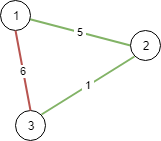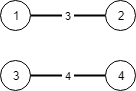## Amazon Online Assessment Questions 2021 OA2 - Min Cost To Add New Roads

There are N cities numbered from 1 to N.

You are given connections, where each connections[i] = [city1, city2, cost] represents the cost to connect city1 and city2together. (A connection is bidirectional: connecting city1 and city2 is the same as connecting city2 and city1.)

Return the minimum cost so that for every pair of cities, there exists a path of connections (possibly of length 1) that connects those two cities together. The cost is the sum of the connection costs used. If the task is impossible, return -1.

Example 1:Input: N = 3, connections = [[1,2,5],[1,3,6],[2,3,1]]
Output: 6
Explanation:
Choosing any 2 edges will connect all cities so we choose the minimum 2.

Example 2:Input: N = 4, connections = [[1,2,3],[3,4,4]]
Output: -1
Explanation:
There is no way to connect all cities even if all edges are used.
Note:

1 <= N <= 10000
1 <= connections.length <= 10000
1 <= connections[i], connections[i] <= N
0 <= connections[i] <= 10^5
connections[i] != connections[i]

Solution:
Try to connect cities with minimum cost, then find small cost edge first, if two cities connected by the edge do no have same ancestor, then union them.
When number of unions equal to 1, all cities are connected.
Time Complexity: O(mlogm + mlogN). sort takes O(mlogm). find takes O(logN). With path compression and unino by weight, amatorize O(1).
Space: O(N).

##### Solve the problem:
Python3
def minCostConnectNodes(N, connections):